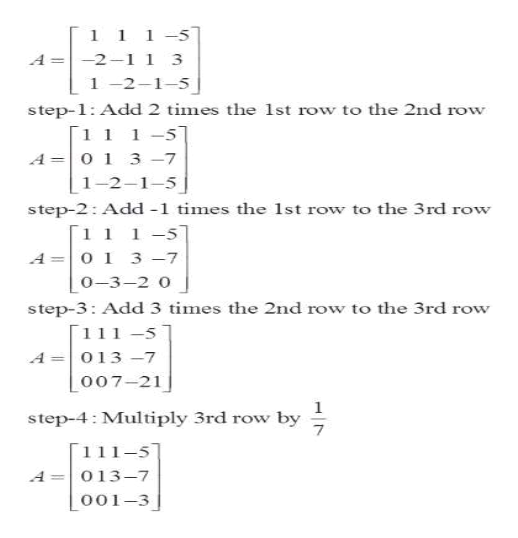# What is the value of x in the system of equations below?x + y + z =-5-2x - y + z = 3x - 2y - z = -5

Question
3 views
 What is the value of x in the system of equations below? x + y + z =-5 -2x - y + z = 3 x - 2y - z = -5
check_circle

Step 1

We are given system of equations

Step 2

Firstly, we will find augmented matrix

Step 3

Now, we can find  reduced row echelon for...help_outlineImage Transcriptionclose[ 1 1 1-5 A =-2-1 i 3 1 -2-1-5 step-1: Add 2 times the 1st row to the 2nd row [1 1 1-5 A O 1 3 -7 1-2-1-5 step-2: Add -1 times the 1st row to the 3rd row [1 1 1-5 A=0 1 3 -7 o-3-2 o step-3: Add 3 times the 2nd row to the 3rd row [1 1 1 -5 A=013 -7 o07-21 1 step-4 Multiply 3rd row by 7 [1 11-51 013-7 A 001-3 fullscreen

### Want to see the full answer?

See Solution

#### Want to see this answer and more?

Solutions are written by subject experts who are available 24/7. Questions are typically answered within 1 hour.*

See Solution
*Response times may vary by subject and question.
Tagged in

### Equations and In-equations